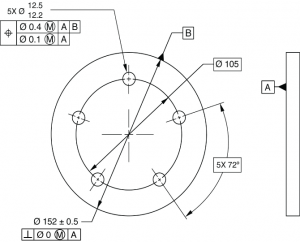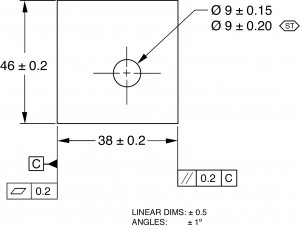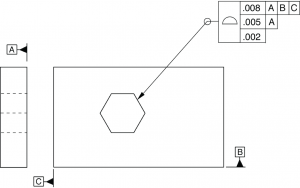# Sample Senior-Level Questions for ASME Certification in GD&T

Several months ago I wrote about the requirements for becoming certified as a “GD&T Professional.”   (View that blog entry here.)    This time, I would like to present some advanced questions.

There are two levels of certification for GD&T Professionals: Technologist and Senior. You do not need to be certified at the Technologist level in order to take the Senior level exam.  But you would need to be sure that you are very familiar with all the tricky nuances of GD&T!  (Plus, you must submit a letter of verification that you have been regularly involved with GD&T for at least five years.)

So the following are a few questions that might be representative of the more difficult stuff.  Answers are at the end, along with the appropriate paragraph number from the 1994 edition of Y14.5…

Sample question #1:

The derived median plane of a feature can best be described as:

a. a theoretically perfect plane through the center of a part’s actual mating envelope

b. a plane that coincides with the centerplane of the true geometric counterpart

c. an imperfect plane passing through center points of all line segments bounded by the feature

d. an imperfect plane representing the average height of a surface plate used to simulate a datum surface

Sample question #2:

Simulating equalized datums may be accomplished by using:

a. pins

b. V-type knife edges

c. V-type planes

d. all of the aboveSample question #3:

For the drawing given above, assume perfect form and perfect orientation. The minimum permissible distance between the outside of the part and the edge of a hole is:

a. 16.6

b. 16.65

c. 16.8

d. 16.95

Sample question #4:

The dimension line of an angle should be:

a. an arc

b. normal to the extension line

c. parallel to the extension line

d. angled to the extension line

Sample question #5:

For the following print, a part of Ø9.17 would be _______ .

a. a good part

c. good, only if statistical data is monitored

d. good only if a stack is calculatedSample question #6:

In the following drawing, the .008, .005, and .002 tolerances control ________ respectively.

a. location & size; orientation; form

b. location & perpendicularity; location; form

c. location; orientation & size; form

d. location & orientation; perpendicularity; size & formHere are the answers:  1=c (paragraph 1.3.15), 2=d (4.6.6), 3=b (4.5.3 and 5.4.1), 4=a (1.7.1.3), 5=c (2.16), 6=d (6.5.9.1)

1.Thank you for sharing the questions. These are tricky, need extra efforts.

2.thanks for sharing…………..

3.thankyou for giving this information can u give more questions on interview point on this topic, it is very helpful to us

4.Senior level examination schedule is for 6 hrs… Could you please tell us what are the question types. only multiple choices are is it some thing that we have to write/ or any face to face interview ? Could any one help…

5.for question 3 i get 16.15 as answer
+151.5/2
-1.0/2
-105/2
-0.7/2
-12.5/2

•Of the numbers you’ve shown, it looks like your error is in the line stating -1.0/2 (I think you meant to write -0.1/2)
But that should be -0.4/2 since we are to use the upper position tolerance, not the lower. This is because we are looking for the position of the hole relative to the part’s outside wall, which is datum feature B.

For everyone’s benefit, here’s one way to do it: First look at the difference in the radii. Take half of the part’s outside diameter (at its smallest) and then subtract half of the basic diameter of the bolt circle. This would be: 151.5/2 – 105/2 = 23.25

Next examine the position tolerance, which we can think of as applying around the axis of the hole: The stated tolerance is a diameter of 0.4, but because of the “M” modifier we know that the 0.4 can grow to 0.7. This is because the hole’s size can be made anywhere from dia 12.2 (MMC) to 12.5 (LMC). Now, since that total position tolerance of 0.7 is a diameter, it means the axis can move 0.35 in any direction. Apply this error to our previous answer: 23.25 – 0.35 = 22.9

Finally we need to account for the physical diameter of the hole. The minimum wall thickness occurs when the hole is at LMC, or dia 12.5. This equates to a radius of 6.25, so we subtract that from our previous answer: 22.9 – 6.25 = 16.65 or answer b.

•I also have the same idea with ARUN PRASAD.

His the second line of formula, -1.0/2, comes from the bonus tolerance (-1.0) at outside 151.5(LMC),

Don’t we need to consider the bonus tolerance of outside diameter at LMC?

•We don’t consider any extra tolerance from datum feature B, because B does not have an “M” modifier in the feature control frame. So while we are to consider the smallest size of B (151.5/2), the geometric tolerance is considering datum B “regardless of material boundary.”

•I think you mentioned 151.2/2 instead of 151.5/2. Its look like typo error. Please correct if I am right,
So it make easy to understand.

•You’re right — I have fixed that in my reply above, in the paragraph starting “for everyone’s benefit.” (The answer was correct, but in that explanatory reply I did have a typo.) Thanks!

•Here is how I worked it out:

152 / 2 = 76 ; Nominal OD radius
105 / 2 = 52.5 ; Nominal ID radius
12.5 / 2 = 6.25 ; Hole radius at MMC

52.5 + 6.25 = 58.75 ; Distance from center to edge of hole at MMC
76 – 58.75 = 17.25 ; Nominal distance from -B- to edge of hole

17.25 – .5 = 16.75 ; Distance minus OD tolerance
16.75 – .1 = 16.65 ; Distance minus Hole position tolerance relative to -A-

•For Ques 3 :
Outer dia : 152-0.5 = 151.5
Gap between two bigger hole : 151.5-105 = 46.5/2 = 23.25
Pin Size : 12.5+0.4+0.3 = 12.9 = 6.6
Required Gap : 23.25-6.6 = 16.65

6.1.RC of Hole = 12.5+0.4+0.3=13.2
2.plus pitch circle dia=105

Add 13.2+105 = 118.2 find max edge of hole

Twist is perfect & Orientation need to consider RFS for outside dia, so Min dia = 151.5

Min wall thick = 151.5-118.2
= 33.3/2
= 16.65

•may i know Why did not you consider bonus tolerance for LMC of outer dia(151.5)

•See my reply to DS.Kim’s comment above (from June 20, 2017). While there is a “bonus” from that outside diameter when calculating its perpendicularity error, that does not affect the position calculation because there is no “M” after datum B in the position callout. (Also note that the question assumed perfect form and perfect orientation, so while the outside diameter’s size can be reduced to 151.5, we assume it stays perpendicular to A.)

7.For question 6
The option c. location; orientation & size; form is right.
Because perpendicularity is also a orientation control, need not to be mentioned again.
Correct me if i am wrong?

•Almost correct… While perpendicularity is an orientation control, it is not the only type of orientation. The other type to think about is rotational orientation. In other words, the hexagon might be perpendicular to A but rotated 10º clockwise. (This would be an orientation error with respect to B and C.)

So the answer is (d) because the first tolerance controls rotational orientation (as well as location), the second tolerance controls perpendicular orientation (also known as perpendicularity), and the last tolerance refines the shape…and the size because it wraps around all sides of the hexagon.

8.Hi All

Let me know who any queries in regards the Senior level exam.

9.John-Paul, it would be interesting how many would figure question 3 if the instruction “assume perfect form and perfect orientation” were omitted.
Regards,
Larry Weber GDTP S-0636

•Indeed, Larry! Actually, that statement was not in our question when we first posted this, and the topic was brought to our attention so we added that condition to the question. Thanks for looking.

10.I recently completed a Blueprint Certification course through the NTMA and the final exam included questions 1-5 listed above. However, they did not include the “assume perfect form and perfect orientation” specification. Would you arrive at a different answer without the addition of that statement?

•Hello Jason — Would the answer be different? Yes. Suppose the outside diameter were oval, or suppose that the outside diameter were tilted with respect to datum A. Then the wall thickness being asked about would not be a consistent answer; with tilt it might be a narrow distance near one face of the part and thicker near the other face.
The question could be solved, but it would be more difficult, and it wouldn’t necessarily be a consistent answer throughout the depth of the part, or consistent around the 5 holes.
PS… thanks for the tip that NTMA is using our questions.

11.John-Paul,

I think that if the instruction in the question was changed from “assume perfect form and perfect orientation” to just “assume perfect form” in question 6, the answer would be 16.15.

The reason: we would need to consider the hole’s Resultant Condition (related to the positional tolerance) with respect to A,B and the OD’s (datum feature B’s) Resultant Condition with respect to A (related to the perpendicularity tolerance).

Am I wrong?

•You’re not wrong. That’s the same idea that I addressed with Jason (see preceding comment), although at the time I didn’t calculate an answer for him with the new assumption in place. I do agree with your answer, because the perpendicularity error could swell to 1.0 mm, allowing for 0.5 tilt (or reduction in our answer) near one face of the part.

The bottom line: The question could have been further clarified if it asked for the minimum constant or consistent wall thickness throughout the depth of the part.

•John-Paul,
Thank you for the affirmation.
Can I additiobaly ask – would the answer change if “assume perfect form” was also removed from the wording of the question? In that case there may be additional variation to be considered because we are talking about both the OD and a hole produced at LMC, so the entire departure from MMC for both features can be translated to form error, then would it be possible for the extreme orientation variation and the extreme form variation to co-exist and reduce the answer even further from 16.15? Thank you again.

•Yes, I agree with you. In fact, this topic recently came up on a discussion forum that I participate in. And I think you’ve participated in that forum as well.
There could be roundness issues with that hole, creating a bump in its outline (if the note about perfect form were not there). So the answer could be further affected by that form error.